# AP Board 4th Class Maths Solutions 7th Lesson Geometry

Andhra Pradesh AP Board 4th Class Maths Solutions 7th Lesson Geometry Textbook Exercise Questions and Answers.

## AP State Syllabus 4th Class Maths Solutions Chapter 7 Geometry

Textbook Page No. 86

Think and Discuss

Question 1.
Does a cone or cylinder have any edges and corners ?
Solution:
Yes, cone has 1 edge and 1 comer.
Yes, cylinder has 2 edges and no comers.

Do this

a) Draw a line to divide the adjacent, rectangles into two equal parts.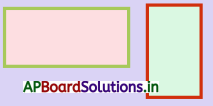Solution: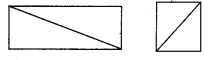b) Name some objects which are in rectangle shape.
Solution:
Book, Brick, Cell phone, Exam pad.

Textbook Page No. 87

Do this

Draw a line to divide the following squares into two equal parts.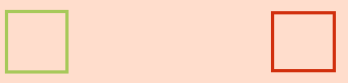Solution: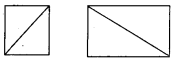Textbook Page No. 89

Do this

a) How many triangles are formed when a square or rectangle is cut diagonally ?
Solution:
When a square or rectangle is cut diagonally two triangles are formed.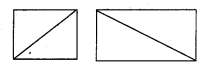b) In a figure, the four sides are 20cm, 16 cm, 20 cm, 16 cm then what is the shape of the object ?
Solution:
Given measurements 20 cm, 16 cm, 20 cm, and 16 cm. Here two sides are equal in measurements. So it is a rectangle.c) In a figure, measurements of four sides are 15 cm each and the adjacent sides are vertical to each other. What is the shape of the object ?
Solution:
Given measurement is 15 cm. All sides are equal and vertical to each other are equal.
∴ It is a square.

Textbook Page No. 90

Do this

Question 1.
Observe the following: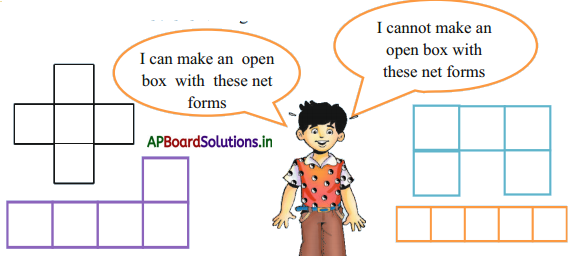Question 2.
Observe the net shapes of the boxes and match them with their 3 – D shapes.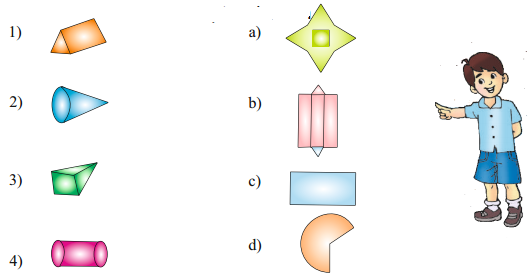Solution:
1 – b
2 – d
3 – a
4 – c

Textbook Page No. 92

Try this

Make some squares, rectangles and triangles with some cool drink straws. Find the perimeter of the shapes.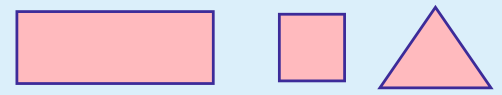Solution:
P = 6m
P = 4m
P = 3m

Exercise – 7.1

Question 1.
The length and breadth of a rectangular field are 60m and 40m respectively. If Somaiah walked around the field, then find the distance covered by him.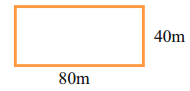Solution:
Length of a rectangular field = 60m
Breadth of a rectangular field = 40 m
Somaiah walked around the field
Distance covered by him = 2(1 + b)
= 2(60 + 40)
= 2 × 100
= 200 mQuestion 2.
Somulu’s site is in square shape. He wanted to construct the compound wall around it. Find the length of the compound wall.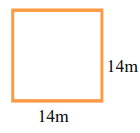Solution:
Length at a square shaped side = 14 cm
He wanted to construct the compound wall around it.
Total length at the compound wall
= 4 side = 4 × 14 = 56 cm

Question 3.
A park is in a triangular shape as shown below. What is the perimeter of the park ?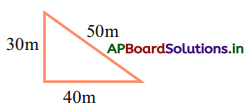Solution:
Park is in a triangular shape.
Length of park sides are 30m, 40m and 50m.
Perimeter of the park = 30 + 40 + 50
= 120 m

4. Find the perimeter of the following figures.

a)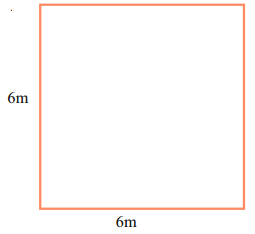Perimeter = ………………. cm
Solution:
From figure, perimeter of figure a
= 6cm + 6cm + 6cm + 6 cm
= 24 cmb)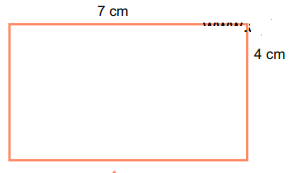Perimeter = ………………. cm
Solution:
From figure, perimeter of figure a
= 4cm + 7cm + 4cm + 7 cm
= 22 cm

c)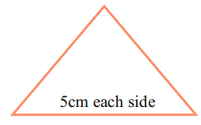Perimeter = ……………… cm
Solution:
From figure, perimeter of figure
= 5cm + 5 cm + 5 cm
= 15 cm

d)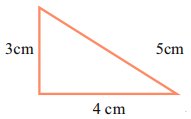Perimeter = ……………… cm
Solution:
Perimeter of the shape a
= 3 cm + 1 cm + 3 cm + 1 cm
= 8 cm

Textbook Page No. 95

Do this

Find the perimeter of the given shapes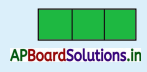Solution: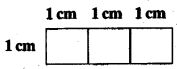∴ Perimeter of the shape
= 3 cm + 1 cm + 3 cm + 1 cm
= 8 cm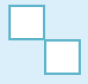Solution: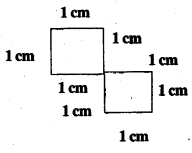∴ Perimeter of the shape
= (1 + 1 + 1 + 1 + 1 + 1 + 1 + 1) cm
= 8 cm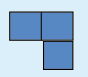Solution: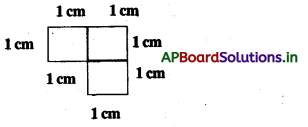∴ Perimeter of the shape
= 2 + 2 + 1 + 1 + 1
= 7 cm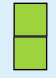Solution: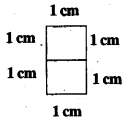∴ Perimeter of the shape
= (1 + 2 + 1 + 2) cm
= 6 cm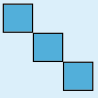Solution: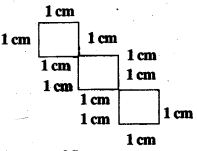∴ Perimeter of the shape
= (1 + 1 + 1 + 1 + 1 + 1 + 1 + 1 + 1 + 1 + 1) cm
= 11 cm

Textbook Page No. 97

Do this

Calculate the area of the shapes formed by colouring three, four and five grids.
Solution:
Area of the shapes formed by colouring
Three grids =3 square units.
Four grids =4 square units.
Five grids = 5 square units.

Try this

Find the area and perimeter of each shaded shape in the pictures. What do you notice?
i)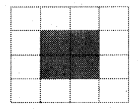This figure occupies 4 grids. Hence its area = 4 square units.
Solution:
This figure occupied 4 grids.
Hence its area = 4 square units.
Perimeter of this figure
= (2 + 2+ 2+ 2) cm
= 8cmii)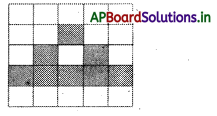This figure occupies 9 grids.
Hence shaded area …………. square units.
Solution:
This figure occupies 9 grids.
Hence shaded area =9 square units.
Perimeter of this figure
= each grid perimeter × 9
= 4cm × 9
= 36 cm

iii)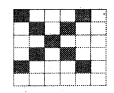This figure occupies ………….. grids.
Hence its area = …………. square units.
Solution:
This figure occupied 8 grids.
Hence shaded area 8 square units.
Perimeter of this figure
= each grid perimeter × 8
= 4 cm × 8
= 32 cm

Exercise – 7.2

Question 1.
On a grid paper, draw different shapes totalling to an area of 8 square units.
Solution: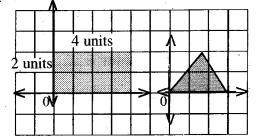Question 2.
Draw a rectangle of 4 units length and 3 units breadth on a grid paper. Also, calculate its area.
Solution: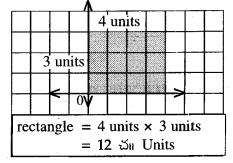Question 3.
Draw a square of side 5 units on a grid paper and calculate them.
Solution: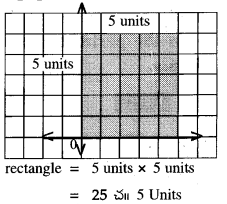Textbook Page No. 100

Do this

a) Draw a few circles using a bangle, plate, bottle cap etc.
Solution: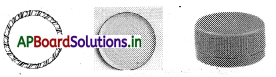b) Draw a circles using one-rupee, two- rupee-five-rupee and ten-rupees coins.
Solution: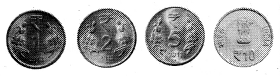Exercise – 7.3

Question 1.
What is the perimeter of the following figures?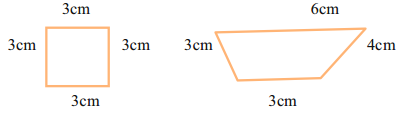Solution:
Perimeter of the given figure
= 3 cm + 3 cm + 3 cm
= 12 cm
Perimeter of the given figure
= 3 cm + 6 cm + 4 cm + 3 cm
= 16 cmQuestion 2.
A piece of land in Simhachalam is in the following shape. Find the length of the fencing wire required to provide fencing around the land.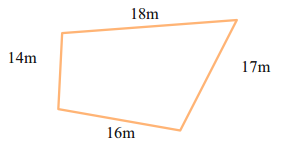Solution:
Length of the fencing wire required to provide fencing around the land
= Perimeter of figure
= 18 m + 17 m + 16 m + 14 m
= 65 m

Question 3.
The perimeter of the following shape is ……………. metres.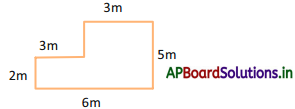Solution:
Perimeter of the given shape
= 3m + 3m + 2m + 5m + 6m
= 19 m

Question 4.
What is the perimeter of the figure?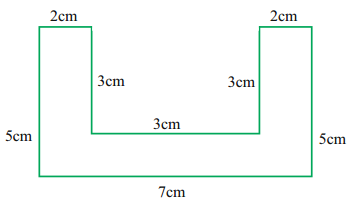Solution:
Perimeter of the figure
= 2cm + cm + 3 cm + 2 cm + 2 cm + 5 cm + 7 cm + 5 cm
= 30 cmQuestion 5.
What is the perimeter and area of figures given below.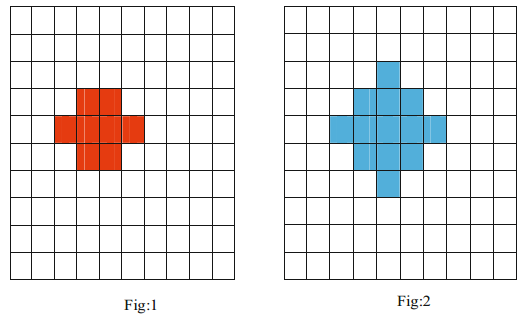Solution:
Perimeter of given figure – 1:
= 2 cm + 1 cm + 1 cm + 1 cm + 1 cm + 1 cm + 1 cm + 2 cm + 1 cm + 1 cm+ 1 cm + 1 cm
= 14 cm

Perimeter of given figure – 2:
= 1 cm + 1 cm + 1 cm + 1 cm + 1 cm+ 1 cm + 1 cm + 1 cm + 1 cm+ 1 cm + 1 cm + 1 cm+ 1 cm+ 1 cm + 1 cm + 1 cm + 1 cm + 1 cm+ 1 cm + 1 cm
= 20 cm

Multiple Choice Questions

Question 1.
Carrot has………….
A) Joker hat
B) Ball
C) Brick
D) Drum
A) Joker hatQuestion 2.
Samosa is in …………. shape.
A) Rectangle
B) Square
C) Circle
D) Triangle
D) Triangle

Question 3.
Shape of Joker cap ……………..
A) Cuboid
B) Cube
C) Square
D) Cone
D) Cone

Question 4.
Carrams board is in ……………….. shape.
A) Rectangle
B) Square
C) Triangle
D) Circle
B) Square

Question 5.
A Rubic cube shape is ……………..
A) Cube
B) Cuboid
C) Cone
D) Square
A) CubeQuestion 6.
Tooth paste box is in ………….. shape.
A) Square
B) Circle
C) Rectangle
D) Triangle
C) Rectangle

Question 7.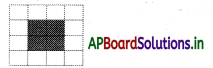Area of shaded region is ……………. sq. units.
A) 9
B) 18
C) 4
D) 10
C) 4

Question 8.
Perimeter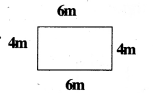of is ………………….. m
A) 20 m
B) 12 m
C) 15 m
D)18 m
A) 20 m

Question 9.
Name of shape of the object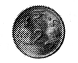A) Square
B) Triangle
C) Circle
D) Rectangle